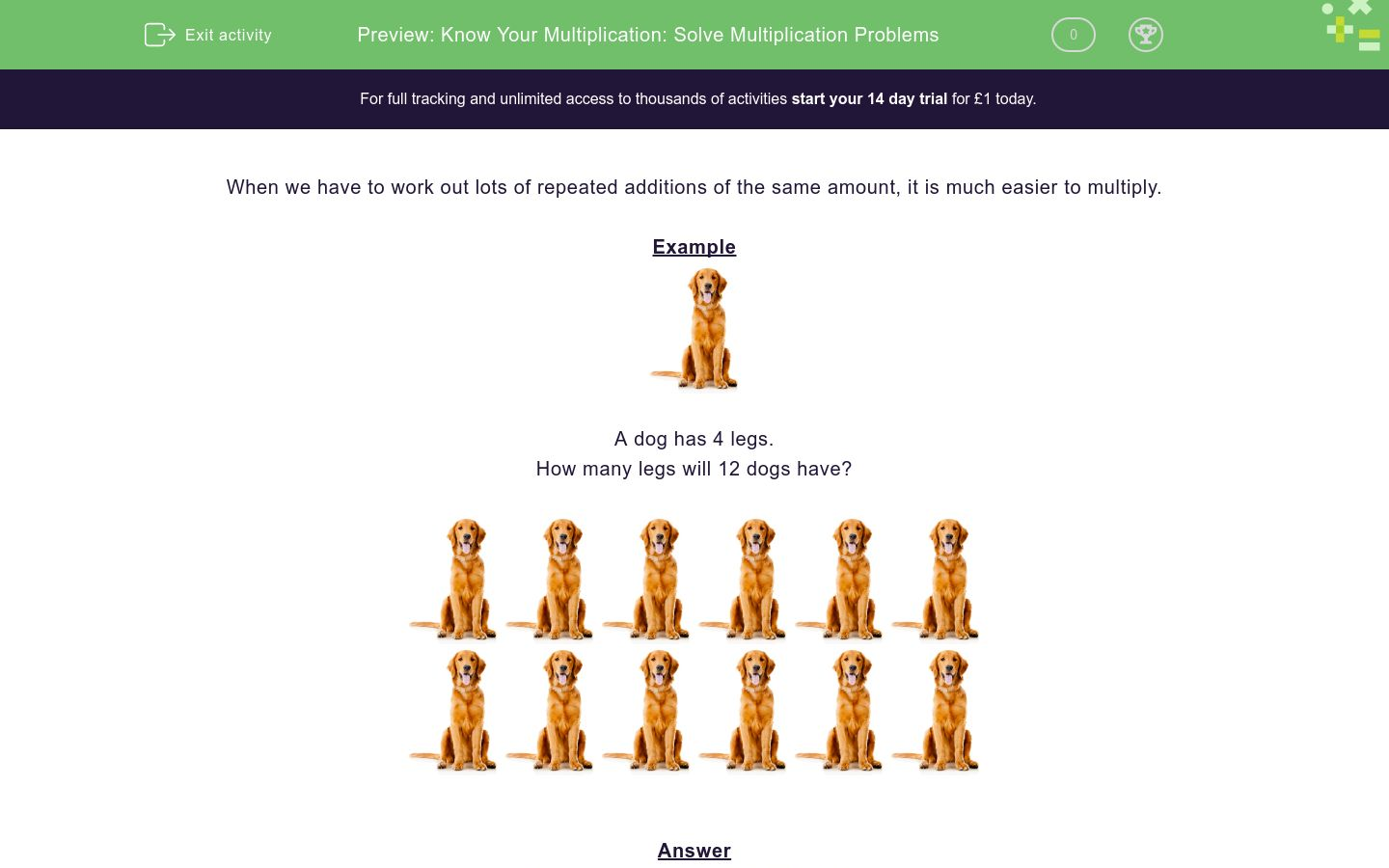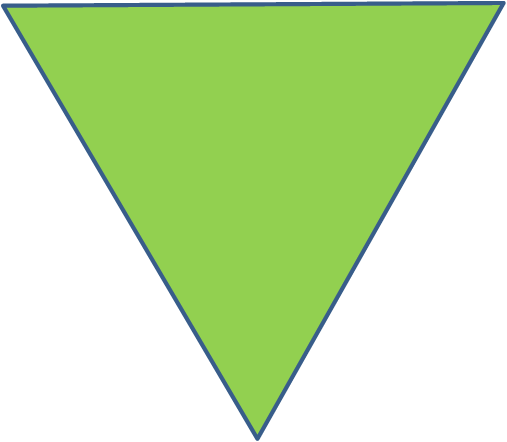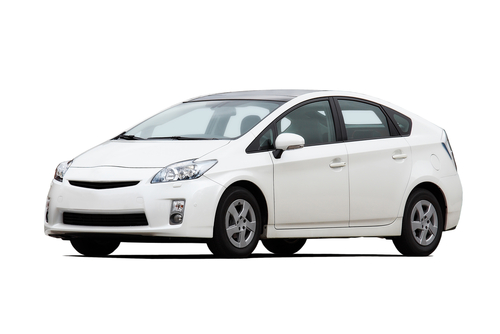# Know Your Multiplication: Solve Multiplication Problems

In this worksheet, students must solve multiplication problems.Key stage:  KS 2

Curriculum topic:   Number: Multiplication and Division

Curriculum subtopic:   Solve Multiplication/Division Problems

Difficulty level:### QUESTION 1 of 10

When we have to work out lots of repeated additions of the same amount, it is much easier to multiply.

ExampleA dog has 4 legs.

How many legs will 12 dogs have?We need to work out  12 lots of 4,

which is quicker than adding 4 + 4 + 4 + 4 + 4 + ... etc. twelve times.

This is   12 × 4.

12 × 4 = 48

12 dogs will have 48 legs.

A horse has 4 legs.

How many legs will 8 horses have?

(Just write the number.)A triangle has 3 sides.

How many sides will 12 triangles have?There are 11 players in a football team.

Each of them has 4 shirts.

How many shirts do they have in total?Isaac has 8 apples.

He cuts each apple into quarters (4 pieces).

How many pieces does he have in total?A tube of tennis balls contains 4 balls.

How many tennis balls does he buy?A car has 4 wheels.

How many wheels will there be on 12 cars?There are 3 lollipops in a bag.

How many lollipops does she buy?Apples cost 8p each.

How much will 12 apples cost in pence?

(Just write the number.)Liz and her 5 friends each write 4 postcards.

How many postcards are written?This flower has 5 petals.

How many petals will there be on 12 similar flowers?• Question 1

A horse has 4 legs.

How many legs will 8 horses have?

(Just write the number.)32
EDDIE SAYS
8 × 4 = 32 legs
• Question 2

A triangle has 3 sides.

How many sides will 12 triangles have?36
EDDIE SAYS
12 × 3 = 36 sides
• Question 3

There are 11 players in a football team.

Each of them has 4 shirts.

How many shirts do they have in total?44
EDDIE SAYS
11 × 4 = 44 shirts
• Question 4

Isaac has 8 apples.

He cuts each apple into quarters (4 pieces).

How many pieces does he have in total?32
EDDIE SAYS
8 × 4 = 32 pieces
• Question 5

A tube of tennis balls contains 4 balls.

How many tennis balls does he buy?20
EDDIE SAYS
5 × 4 = 20 balls
• Question 6

A car has 4 wheels.

How many wheels will there be on 12 cars?48
EDDIE SAYS
12 × 4 = 48 wheels
• Question 7

There are 3 lollipops in a bag.

How many lollipops does she buy?15
EDDIE SAYS
5 × 3 = 15 lollipops
• Question 8

Apples cost 8p each.

How much will 12 apples cost in pence?

(Just write the number.)96
EDDIE SAYS
12 × 8 = 96 p
• Question 9

Liz and her 5 friends each write 4 postcards.

How many postcards are written?24
EDDIE SAYS
6 × 4 = 24 postcards
• Question 10

This flower has 5 petals.

How many petals will there be on 12 similar flowers?60
EDDIE SAYS
12 × 5 = 60 petals
---- OR ----

Sign up for a £1 trial so you can track and measure your child's progress on this activity.

### What is EdPlace?

We're your National Curriculum aligned online education content provider helping each child succeed in English, maths and science from year 1 to GCSE. With an EdPlace account you’ll be able to track and measure progress, helping each child achieve their best. We build confidence and attainment by personalising each child’s learning at a level that suits them.

Get started